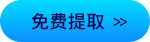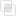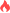java动态规划是什么2021-04-13 16:06:44298浏览 · 0收藏 · 0评论1、说明

2、使用流程

3、实例

```public class dpSolution {
static int getValue(int[] values, int rodLength) {
int[] subSolutions = new int[rodLength + 1];

for (int i = 1; i <= rodLength; i++) {
int tmpMax = -1;
for (int j = 0; j < i; j++)
tmpMax = Math.max(tmpMax, values[j] + subSolutions[i - j - 1]);
subSolutions[i] = tmpMax;
}
return subSolutions[rodLength];
}

public static void main(String[] args) {
int[] values = new int[]{3, 7, 1, 3, 9};
int rodLength = values.length;

System.out.println("Max rod value: " + getValue(values, rodLength));
}
}```java动态代理如何实现？java懒惰评估如何实现1026

java尾部递归如何使用687

python如何判断文件夹内的重复图片1077